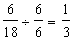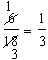# Cancel fractions

## Write in Lowest Terms with Canceling Demonstration

A CANCELING DEMONSTRATION
1

# CANCEL FRACTIONS WITH CIRCLE MODELS

This movie uses circles to demonstrate how to renamed to lowest terms with canceling.

The following equivalent fractions were made from Rename Lowest with Canceling :When the program starts, you will be shown a fraction in higher terms. In the example above, the first fraction has a numerator of 6 and a denominator of 18. The largest number that divides evenly into both 6 and 18 is 6. Six (6) is the greatest common factor of 6 and 18.

This program illustrates how a line is drawn through the numerator to show division of the numerator by the greatest common factor.

The numerator of the second fraction is 1 because 6 divided by 6 is 1.

Then a line is drawn through the denominator to show division of the denominator by the greatest common factor. The denominator of the second fraction is 3 because 18 divided by 6 is 3.

Written out, the example would look like this:Canceling is a short-cut way of writing the above example. Writing in lowest terms is also known as simplifying the fraction.